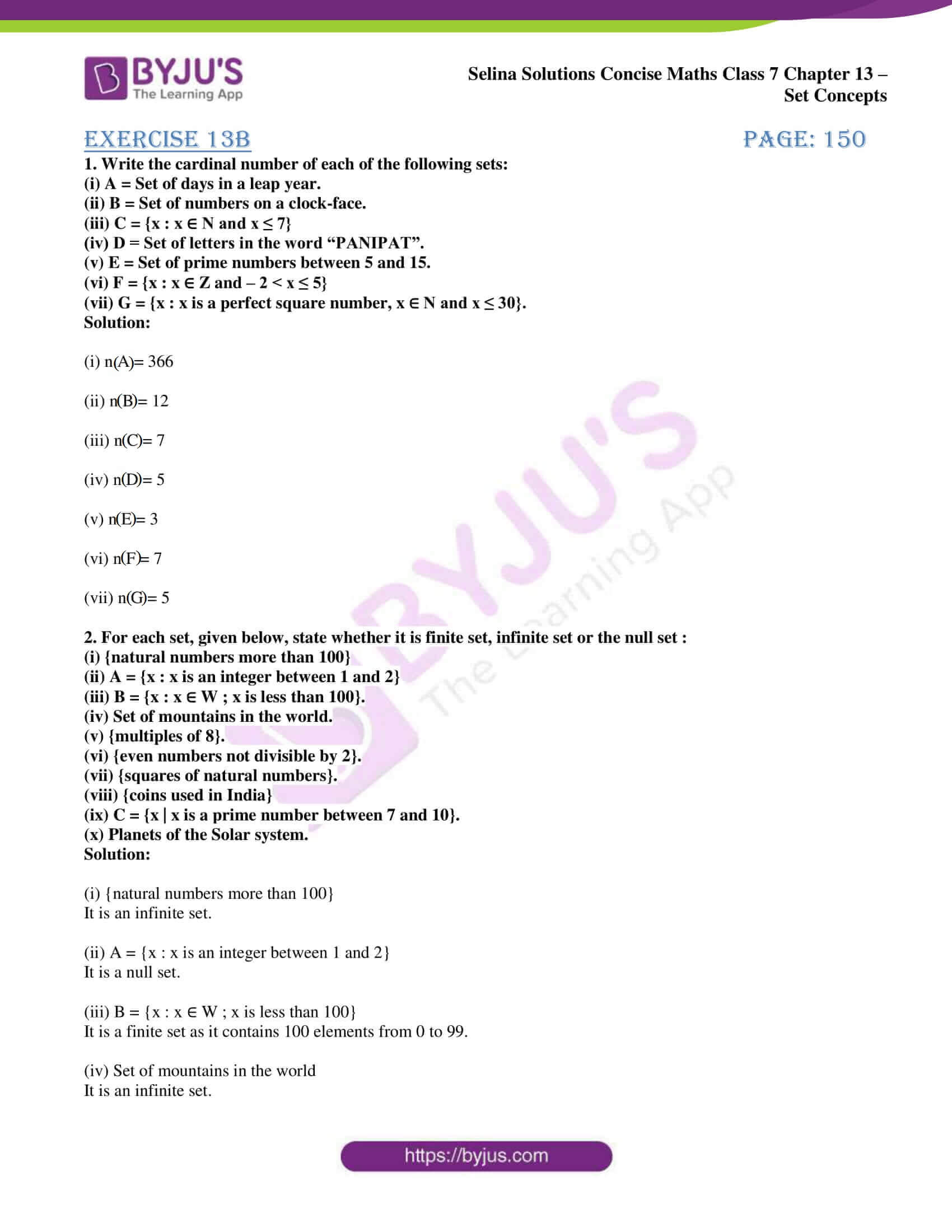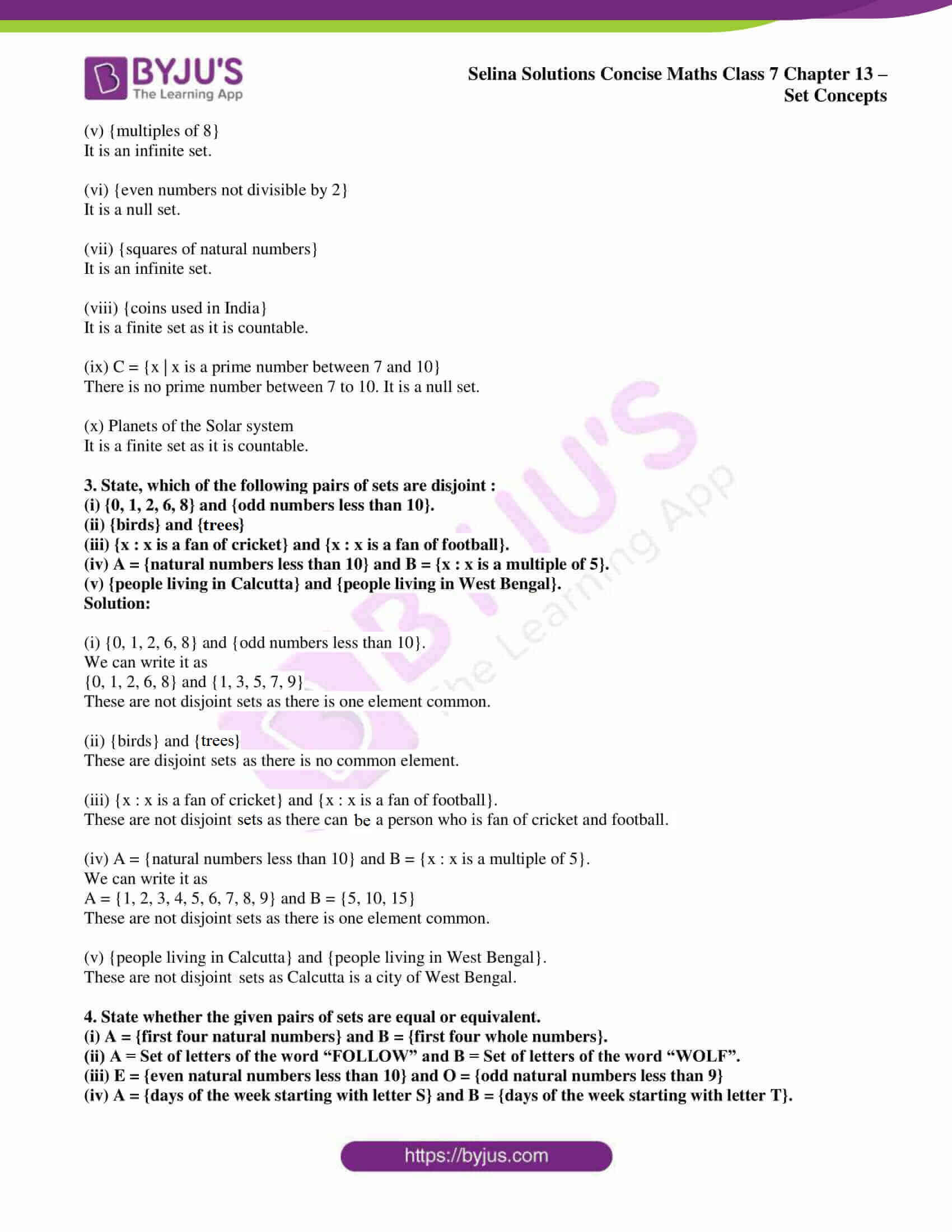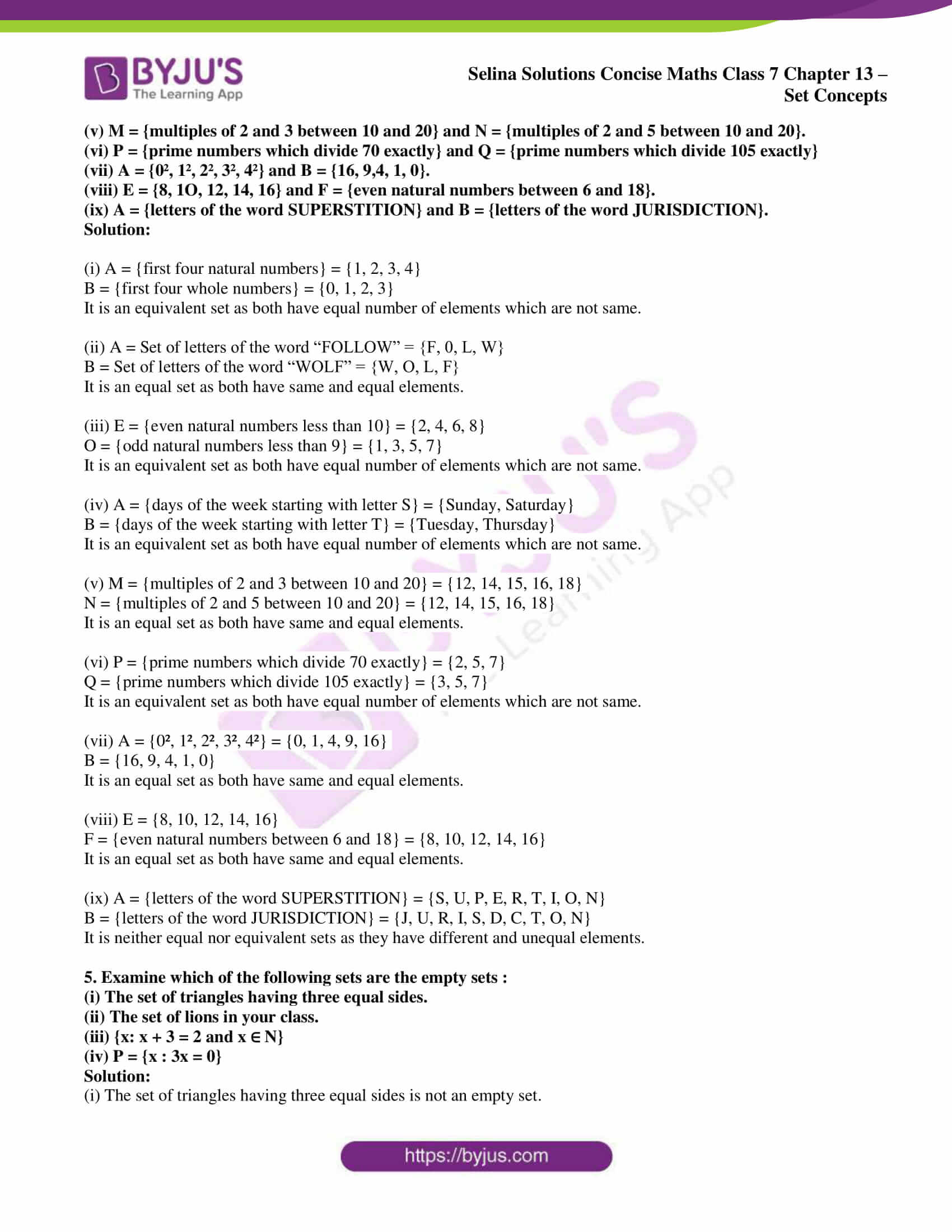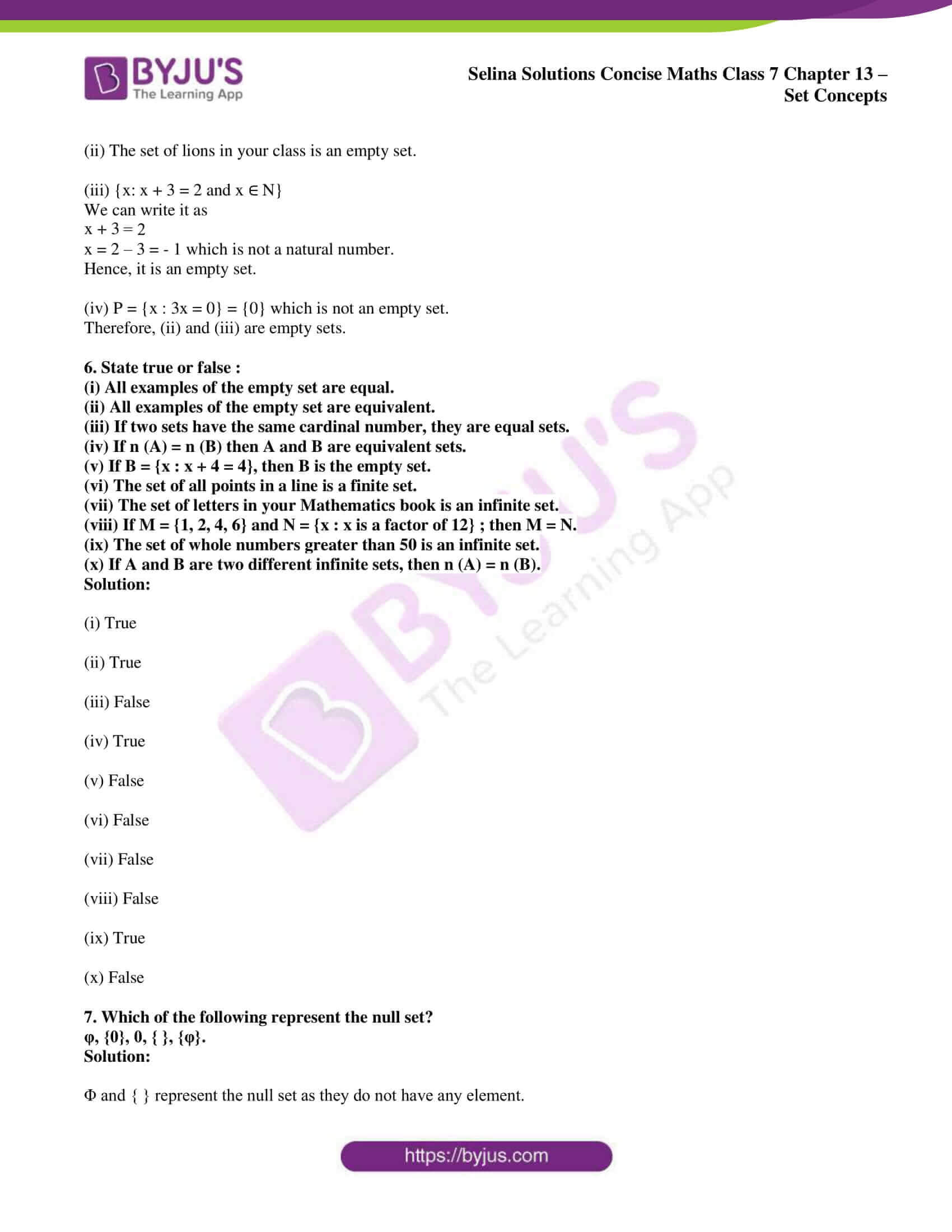# Selina Solutions Concise Maths Class 7 Chapter 13: Set Concepts Exercise 13B

Selina Solutions Concise Maths Class 7 Chapter 13 Set Concepts Exercise 13B help students understand the concept of cardinal number and the types of sets. The number of elements in a set is known as the cardinal number. The various types of sets are explained in simple language, with numerous examples to boost the understanding abilities of students. The solutions PDF can be referred by the students to score well in the annual exam. For a better hold on these concepts, students can download Selina Solutions Concise Maths Class 7 Chapter 13 Set Concepts Exercise 13B free PDF, from the links provided below.

## Selina Solutions Concise Maths Class 7 Chapter 13: Set Concepts Exercise 13B Download PDF### Access other exercises of Selina Solutions Concise Maths Class 7 Chapter 13: Set Concepts

Exercise 13A Solutions

Exercise 13C Solutions

Exercise 13D Solutions

### Access Selina Solutions Concise Maths Class 7 Chapter 13: Set Concepts Exercise 13B

#### Exercise 13B page: 150

1. Write the cardinal number of each of the following sets:
(i) A = Set of days in a leap year.
(ii) B = Set of numbers on a clock-face.
(iii) C = {x : x ∈ N and x ≤ 7}
(iv) D = Set of letters in the word “PANIPAT”.
(v) E = Set of prime numbers between 5 and 15.
(vi) F = {x : x ∈ Z and – 2 < x ≤ 5}
(vii) G = {x : x is a perfect square number, x ∈ N and x ≤ 30}.

Solution:

(i) n (A) = 366

(ii) n (B) = 12

(iii) n (C) = 7

(iv) n (D) = 5

(v) n (E) = 3

(vi) n (F) = 7

(vii) n (G) = 5

2. For each set, given below, state whether it is finite set, infinite set or the null set :
(i) {natural numbers more than 100}
(ii) A = {x : x is an integer between 1 and 2}
(iii) B = {x : x ∈ W ; x is less than 100}.
(iv) Set of mountains in the world.
(v) {multiples of 8}.
(vi) {even numbers not divisible by 2}.
(vii) {squares of natural numbers}.
(viii) {coins used in India}
(ix) C = {x | x is a prime number between 7 and 10}.
(x) Planets of the Solar system.

Solution:

(i) {natural numbers more than 100}

It is an infinite set.

(ii) A = {x : x is an integer between 1 and 2}

It is a null set.

(iii) B = {x : x ∈ W ; x is less than 100}

It is a finite set as it contains 100 elements from 0 to 99.

(iv) Set of mountains in the world

It is an infinite set.

(v) {multiples of 8}

It is an infinite set.

(vi) {even numbers not divisible by 2}

It is a null set.

(vii) {squares of natural numbers}

It is an infinite set.

(viii) {coins used in India}

It is a finite set as it is countable.

(ix) C = {x | x is a prime number between 7 and 10}

There is no prime number between 7 to 10. It is a null set.

(x) Planets of the Solar system

It is a finite set as it is countable.

3. State, which of the following pairs of sets are disjoint :
(i) {0, 1, 2, 6, 8} and {odd numbers less than 10}.
(ii) {birds} and {trees}
(iii) {x : x is a fan of cricket} and {x : x is a fan of football}.
(iv) A = {natural numbers less than 10} and B = {x : x is a multiple of 5}.
(v) {people living in Calcutta} and {people living in West Bengal}.

Solution:

(i) {0, 1, 2, 6, 8} and {odd numbers less than 10}.

We can write it as

{0, 1, 2, 6, 8} and {1, 3, 5, 7, 9}

These are not disjoint sets as there is one element common.

(ii) {birds} and {trees}

These are disjoint sets as there is no common element.

(iii) {x : x is a fan of cricket} and {x : x is a fan of football}.

These are not disjoint sets as there can a person who is a fan of cricket and football.

(iv) A = {natural numbers less than 10} and B = {x : x is a multiple of 5}.

We can write it as

A = {1, 2, 3, 4, 5, 6, 7, 8, 9} and B = {5, 10, 15}

These are not disjoint sets as there is one element common.

(v) {people living in Calcutta} and {people living in West Bengal}.

These are not disjoint sets as Calcutta is a city of West Bengal.

4. State whether the given pairs of sets are equal or equivalent.
(i) A = {first four natural numbers} and B = {first four whole numbers}.
(ii) A = Set of letters of the word “FOLLOW” and B = Set of letters of the word “WOLF”.
(iii) E = {even natural numbers less than 10} and O = {odd natural numbers less than 9}
(iv) A = {days of the week starting with letter S} and B = {days of the week starting with letter T}.
(v) M = {multiples of 2 and 3 between 10 and 20} and N = {multiples of 2 and 5 between 10 and 20}.
(vi) P = {prime numbers which divide 70 exactly} and Q = {prime numbers which divide 105 exactly}
(vii) A = {0², 1², 2², 3², 4²} and B = {16, 9,4, 1, 0}.
(viii) E = {8, 1O, 12, 14, 16} and F = {even natural numbers between 6 and 18}.
(ix) A = {letters of the word SUPERSTITION} and B = {letters of the word JURISDICTION}.

Solution:

(i) A = {first four natural numbers} = {1, 2, 3, 4}

B = {first four whole numbers} = {0, 1, 2, 3}

It is an equivalent set as both have equal number of elements which are not same.

(ii) A = Set of letters of the word “FOLLOW” = {F, 0, L, W}

B = Set of letters of the word “WOLF” = {W, O, L, F}

It is an equal set as both have same and equal elements.

(iii) E = {even natural numbers less than 10} = {2, 4, 6, 8}

O = {odd natural numbers less than 9} = {1, 3, 5, 7}

It is an equivalent set as both have equal number of elements which are not same.

(iv) A = {days of the week starting with letter S} = {Sunday, Saturday}

B = {days of the week starting with letter T} = {Tuesday, Thursday}

It is an equivalent set as both have equal number of elements which are not same.

(v) M = {multiples of 2 and 3 between 10 and 20} = {12, 14, 15, 16, 18}

N = {multiples of 2 and 5 between 10 and 20} = {12, 14, 15, 16, 18}

It is an equal set as both have same and equal elements.

(vi) P = {prime numbers which divide 70 exactly} = {2, 5, 7}

Q = {prime numbers which divide 105 exactly} = {3, 5, 7}

It is an equivalent set as both have equal number of elements which are not same.

(vii) A = {0², 1², 2², 3², 4²} = {0, 1, 4, 9, 16}

B = {16, 9, 4, 1, 0}

It is an equal set as both have same and equal elements.

(viii) E = {8, 10, 12, 14, 16}

F = {even natural numbers between 6 and 18} = {8, 10, 12, 14, 16}

It is an equal set as both have same and equal elements.

(ix) A = {letters of the word SUPERSTITION} = {S, U, P, E, R, T, I, O, N}

B = {letters of the word JURISDICTION} = {J, U, R, I, S, D, C, T, O, N}

It is neither equal nor equivalent sets as they have different and unequal elements.

5. Examine which of the following sets are the empty sets :
(i) The set of triangles having three equal sides.
(ii) The set of lions in your class.
(iii) {x: x + 3 = 2 and x ∈ N}
(iv) P = {x : 3x = 0}

Solution:

(i) The set of triangles having three equal sides is not an empty set.

(ii) The set of lions in your class is an empty set.

(iii) {x: x + 3 = 2 and x ∈ N}

We can write it as

x + 3 = 2

x = 2 – 3 = – 1 which is not a natural number.

Hence, it is an empty set.

(iv) P = {x : 3x = 0} = {0} which is not an empty set.

Therefore, (ii) and (iii) are empty sets.

6. State true or false :
(i) All examples of the empty set are equal.
(ii) All examples of the empty set are equivalent.
(iii) If two sets have the same cardinal number, they are equal sets.
(iv) If n (A) = n (B) then A and B are equivalent sets.
(v) If B = {x : x + 4 = 4}, then B is the empty set.
(vi) The set of all points in a line is a finite set.
(vii) The set of letters in your Mathematics book is an infinite set.
(viii) If M = {1, 2, 4, 6} and N = {x : x is a factor of 12} ; then M = N.
(ix) The set of whole numbers greater than 50 is an infinite set.
(x) If A and B are two different infinite sets, then n (A) = n (B).

Solution:

(i) True

(ii) True

(iii) False

(iv) True

(v) False

(vi) False

(vii) False

(viii) False

(ix) True

(x) False

7. Which of the following represent the null set?
φ, {0}, 0, { }, {φ}.

Solution:

Φ and { } represent the null set as they do not have any element.